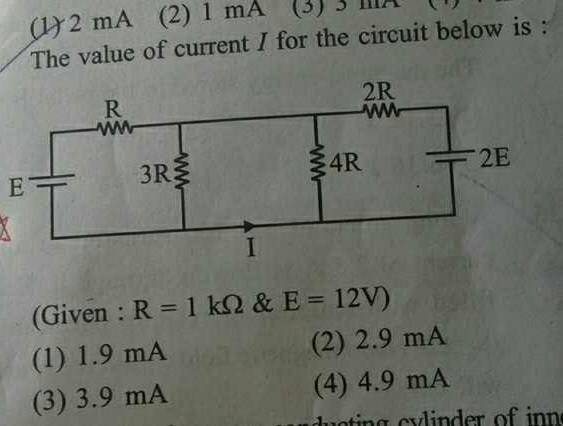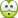# Current flowing in a branch in a circuit

## Homework Statement## The Attempt at a Solution

For calculation sake I will consider R = 1Ω . Final answer will be in mA instead of A .

The middle two resistors 3R and 4R are in parallel , so I consider their equivalent resistance 12R/7 in the middle branch .

In the left part of the circuit , suppose i1 current flows in R .

In the right part of the circuit , suppose i2 current flows in 2R .

Current flowing in the middle 12R/7 resistor will be i

Using KCL i = (i1+i2)

Writing KVL in the left loop ,
12 - i1 - 12i/7 = 0

Writing KVL in the right loop ,
24 - 2i2- 12i/7 = 0

Solving for i , i = 6.72

This is the sum of the currents that will flow in the middle 3R and 4R resistors .Since they are in parallel , current will be divided in the ratio 4:3 .This means (1/7)th of the current i =6.72 flows in the middle lower branch ( the one that is asked in the question )

This gives 0.96 which is incorrect .

What is the mistake ?

#### Attachments

cnh1995
Homework Helper
Gold Member
I believe node voltage method is an easier way to do this. Call the middle node voltage V and write KCL at that node. Assume the negatives of the batteries to be the ground node.

I believe node voltage method is an easier way to do this. Call the middle node voltage V and write KCL at that node. Assume the negatives of the batteries to be the ground node.

I will do as you suggest . But for now , please help me identify the mistake in the OP .

cnh1995
Homework Helper
Gold Member
This means (1/7)th of the current i =6.72 flows in the middle lower branch ( the one that is asked in the question )
How? I see you have assumed I=I-I.
Is that correct?

•Jahnavi
How? I see you have assumed I=I-I.
Is that correct?

No .

It should be (4/7)i - i1 . But this gives 3.36

I believe node voltage method is an easier way to do this. Call the middle node voltage V and write KCL at that node. Assume the negatives of the batteries to be the ground node.

I tried your approach . This also gives 3.36.

cnh1995
Homework Helper
Gold Member
I tried your approach . This also gives 3.36 A .
Right. I get the same answer.

•Jahnavi
ehild
Homework Helper
No .

It should be (4/7)i - i1 . But this gives 3.36
The same as I got.

•Jahnavi
Is it only me who is not able to see 3.36mA in any of the given options?

cnh1995
Homework Helper
Gold Member
Is it only me who is not able to see 3.36mA in any of the given options?
It's not the first time that the options are incorrect.•Jahnavi
By the way , I agree that your approach is much simplerand most probably the paper setter expects us to apply the Node Voltage method .

Assume the negatives of the batteries to be the ground node.

This is quite helpful . What if the polarity of one of the batteries is reversed ?

Do you still think that node voltage method will still be more efficient and simpler considering that now there will be two equations to deal with ?

cnh1995
Homework Helper
Gold Member
Do you still think that node voltage method will be still more efficient and simpler considering that now there will be two equations to deal with ?
Yes. No matter what the polarities are, there are still only 4 nodes, one of which is the ground node and you know two node voltages. So you end up with a single equation with a single variable.
In general, you can assume any node to be the ground node. As long as you are consistent with signs in KCL, you are fine.

•Jahnavi
Thank you so much !

Last edited: